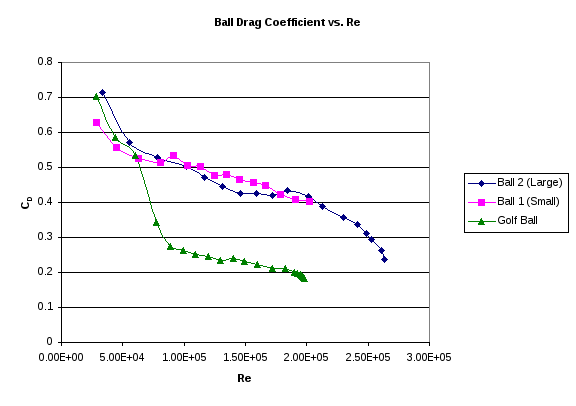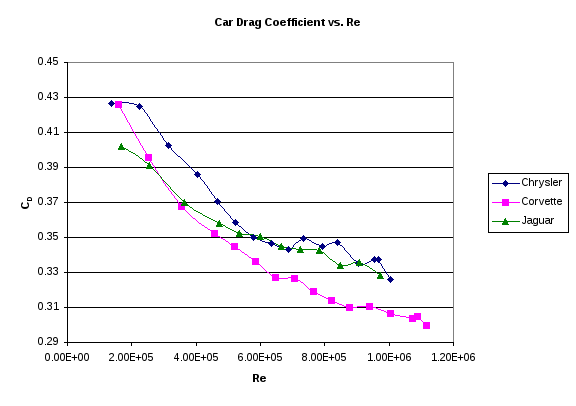# Katia Hristova, Scott Kahre, Andrew Bussey

 Date 20.05.2018 Size 44.22 Kb.
Wind Tunnel Experiment

Lab 1

ChE 341

Katia Hristova, Scott Kahre, Andrew Bussey

There were three main goals of this experiment. First, we wanted to compare the drag coefficient of two differently sized smooth spheres in a flowing air stream. Secondly, we compared the drag coefficients of smooth spheres to that of a rough sphere (a golf ball in this case). Finally, several model vehicles were tested to determine their coefficients of drag at a range of Reynolds numbers. All of this testing was performed in a wind tunnel while controlling air velocity and measuring drag force.Our graph of drag coefficient vs. Reynolds number (Fig. 1) for two smooth spheres follows the same overall trend as that published in the textbook. But, on close inspection, it can be seen that the drag coefficient does not have a positive slope at Reynolds numbers just below 1x105 like the one in the textbook. For both spheres, at Re of approximately 1x105, CD is approximately .51. This compares favorably to the textbook value of CD (.5) at the same Reynolds number. But, at lower Reynolds numbers, our data tracked slightly higher than the published smooth sphere graph. This could be due to the slight roughness of the wood grain on the balls.

The transition to turbulent boundary layer occurred for the large sphere at about Re = 2x105. The maximum speed of the wind tunnel was too low for the small sphere’s boundary layer to transition to turbulent. Our text reports that the transition to turbulent boundary layer for smooth spheres should occur at about Re = 2x105. Thus, our data agrees well with previous experiments for turbulent boundary layer transitions.

Discrepancies between our data and previously obtained results could be explained by several factors. The slight roughness of the wood was mentioned above. Also, the mounting apparatus for the balls in the tunnel had a rather large metal bar connected to the rear of the sphere. This bar could have had a streamlining effect that would reduce the drag. Also, of course, there was drift in the speed and drag sensing equipment that most likely introduced a small error.

For the golf ball, the plot is essentially the same as the smooth spheres for Reynolds numbers up to about 6.5x104, at which point the transition to turbulent boundary layer occurred. At the transition, there was a sharp drop in drag coefficient. At Reynolds numbers above about 8.5x104 the slope of the golf ball’s graph is approximately the same as that of the smooth balls, but drag coefficient is approximately .25 less than the smooth spheres. The reason the transition to turbulent boundary layer occurs at such a low Reynolds number is that the dimples on the surface of the golf ball disrupted the flow at a much lower speed. The dimples create tiny eddies in the boundary layer that allow momentum transfer perpendicular to the flow.If the fuel mileage of a vehicle is directly proportional to its drag coefficient, our results would suggest that the Corvette should get the best fuel economy, followed by the Jaguar, with the Chrysler getting the worst mileage. This assumption is based on the idea that drag is the only load placed on an engine during driving and all engines have the same thermodynamic efficiency.

 Highway Fuel Economy (mi/gal) Engine Size / Cyl. Chrysler 29 2.4L / 4 cyl. Corvette 28 5.7L / 8 cyl. Jaguar 28 2.5L / 6 cyl.

The table above shows the actual highway fuel economy of the three vehicles as reported by the U.S. Department of Energy’s online Fuel Economy Guide. As you can see, the three vehicles have nearly the same fuel economy, even though their drag coefficients vary significantly. So, clearly, the assumption made above was incorrect. This is because engine size and number of cylinders also plays a major role in the efficiency of the engine such that the larger an engine is, the less efficient it is. For the three cars in our experiment, the engine size happens to follow the opposite trend as the drag coefficient. So, in the Corvette, the low drag coefficient is offset by the large engine size, and in the Chrysler, the large drag coefficient is offset by the small engine. The Jaguar is in the middle on both drag coefficient and engine size.

When testing the model for scale speeds, the Reynolds number must be equal for the model and the full scale vehicle. The maximum speed of the wind tunnel we used was approximately 150 mi/hr. Since the car models were 1/18th scale, and the viscosity of the air was the same as that found in real driving, the scale velocity was only 1/18 as much as our model test. That means that the scale velocity was only about 8.33 mi/hr (really slow). In order to test the model for a scale speed of 55 mi/hr, the wind tunnel would have to run at approximately 990 mi/hr. This is supersonic speed, so a more practical method would be to test the model in a fluid with a higher viscosity.

The boundary layer thickness along the floor of the wind tunnel could possibly affect the results if it is comparable to the height of the car. The car with the lowest height was the Corvette, so it will be considered as a “worst case scenario”. At wind speed of 15.75 m/s, the boundary layer at the front of the car was .0092 m thick. This is about 13% of the car’s height. At 69.9 m/s, the boundary layer thickness was .0068 m. This is only 9.7 % of the total car height. Thus, since most of the car was above the boundary layer, there was only a small error introduced by using the stationary model in moving air instead of the moving model in stationary air. So, the drag force in our experiment was only slightly lower (due to the lower velocity in the boundary layer) than what would be found in a moving model experiment.

Overall, the results of the wind tunnel experiment were as expected. The drag coefficient vs. Reynolds number graphs for the smooth spheres were nearly identical to those found in literature. Also, the golf ball exhibited a transition to turbulent boundary layer at a low Reynolds number as expected. Finally, the drag coefficients for the cars were as expected, based on the height and shape of the cars. Unfortunately, the drag coefficients did not predict the fuel economies because the engines were not equivalent. The boundary layer on the wind tunnel floor was small compared to the height of the cars, so it did not affect the results very strongly.